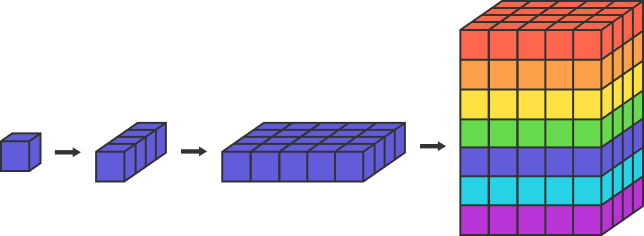# Rainbow BuildingYou are building a rainbow building from $N$ cubic unit blocks.

First, you put together 3 cubes each to form a group of $1\times 3$ columns and discard the remaining cubes.

Then, you put together 5 columns each to form a group of $3\times 5$ cubic bases and discard the remaining columns, as before.

Finally, you stack 7 bases over one another to build the desired $3\times 5\times 7$ cuboid structure, as shown above, and discard all the other bases, as usual.

If there are a total of 52 discarded cubes, and $N$ is a multiple of 11, what is the least possible value of $N?$

×